### Home > CALC > Chapter 5 > Lesson 5.2.5 > Problem5-103

5-103.
1. FUNKY FUNCTIONS REVISITED Homework Help ✎

1. Graph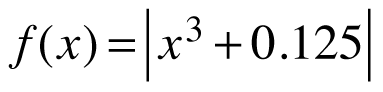and rewrite f(x) as a piecewise function.

2. Zoom in at x = −0.5 on your graphing calculator and carefully examine the curve. Does f(x) appear differentiable at x = −0.5? Why or why not?

3. To confirm whether or notis differentiable at x = −0.5, we need to examine f ′(x). Use the piecewise function from part (a) to find f ′(x) for x ≠ −0.5.

4. Does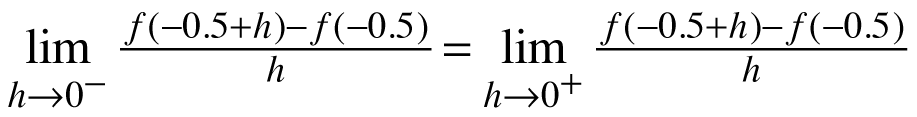? State a conclusion.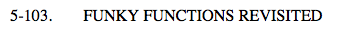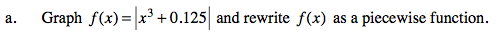There will be two pieces. The graph indicates that x = −0.5 is the boundary point.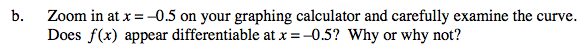Does the slope from the left of x = −0.5 appear to agree with the slope from the right?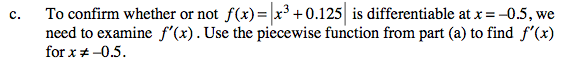Find the derivative of each piece of your piecewise function.
Then evaluate these limits. Do they agree?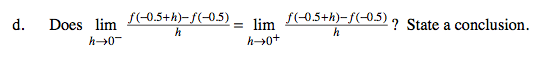Notice Hana's definition of the derivative! This question is asking f '(−0.5) from the left agrees with f '(−0.5) from the right.
Looking at the graphs, do the slopes appear to agree from both sides of −0.5?

Use the eTool below to help solve the problem.
Click the link at right for the full version of the eTool: Calc 5-103 HW eTool